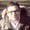# A Smooth Refresher on Python's TuplesThis post is part of a series called A Smooth Refresher To Python.
A Smooth Refresher on Python's Dictionaries
A Smooth Refresher on Python's Conditional Statements

In my previous refreshers, which you can access from the series navigation links at the top of this article, I talked about two important Python concepts you need to grasp in order to move forward in your Python learning journey.

This tutorial is a continuation of the Python refresher series, and today I will be talking about Tuples. That way, you will have three important concepts in your pocket and will be ready to dig deeper in the Python language.

So, let's go ahead and move directly to this interesting topic of Tuples.

If you understood Lists, Tuples will be very simple to grasp, because they are similar to Lists except for two main differences:

1. Tuples are immutable, so once you create a Tuple, you cannot change its content or even its size, unless you make a copy of that Tuple.
2. They are written in parentheses ( ) rather than in square brackets [ ]

Thus, as you can guess, Tuples consist of a set of ordered objects which can be of any type (i.e. Strings, Lists, Dictionaries, Tuples, etc.), and are accessed by an index (offset), as opposed to Dictionaries where items are accessed by key. It is important to note here that Tuples store references to the objects they contain.

Before moving to some Tuple operations, let's see what a simple Tuple looks like:

tup = (1)

This is a simple Tuple that contains one item, an integer value. The output of this Tuple will be 1.

Another example of a Tuple with three items of different object types is as follows:

tup = (31,'abder',4.0)

The output for the above statement is:

(31, 'abder', 4.0)

You can even write the above Tuple without parentheses as follows:

tup = 31,'abder',4.0

Very flexible, isn't it?

As a final example, let's see how a nested Tuple would look:

nested_tuple = ('ID', ('abder', 1234))

## Tuple Operations

Let's now walk through some Tuple operations.

### Concatenation

Concatenation is the combination of Tuples together. Say that we have the following two Tuples:

tuple1 = (1,2,3,4,5)

tuple2 = (6,7,8,9,10)

In order to concatenate tuple1 and tuple2, we simply type:

tup = tuple1 + tuple2

Notice that we used the + operator to perform the concatenation. This will result in the following output:

(1, 2, 3, 4, 5, 6, 7, 8, 9, 10)

### Repetition

Tuple repetition is simply carried out using the * operator. If we want to repeat tuple1 three times, we do the following:

tuple1 * 3

The result of this statement is:

(1, 2, 3, 4, 5, 1, 2, 3, 4, 5, 1, 2, 3, 4, 5)

### Membership

To check the membership of some item in the Tuple, we use in, as follows:

7 in tuple1

This will return False since 7 doesn't belong to tuple1.

### Search

In order to indicate where some item is located in the Tuple, we use index. For instance, if we want to find the location of the item 5 in tuple1, we do the following:

tuple1.index(5)

In this case, the return value will be 4, which is the location of the item 5.

### Count

A nice operation in Tuples is counting the number of times an element exists in the Tuple. Say we have the following Tuple:

tuple3 = (65,67,5,67,34,76,67,231,98,67)

If we want to see how many times 67 exists in tuple3, we simply do the following:

tuple3.count(67)

The result for this statement should be 4 times.

### Indexing

Indexing is the process of accessing a Tuple element by index (subscript). If we want to access the fifth index in tuple3, we do the following:

tuple3

which will return 34.

An index can also be negative, that is, counting will start from the right of the Tuple. Thus, the result of tuples3[-6] will be 34, provided that the range of the negative indices in tuples3 is [-1,-10]

What if you chose an index out of this range? Like tuples3[-11] for instance? This is what you would get in this case:

Traceback (most recent call last):

File "tuple.py", line 2, in <module>

print tuple3[-11]

IndexError: tuple index out of range

Notice that negative indices start from -1. So, if you typed the index as -0, this is the same as the index 0. Thus, tuples3[-0] will return 65.

### Slicing

Unlike indexing, which provides us with one element as a result, slicing provides us with a sequence of elements. An example of slicing is the following statement:

tuples3[2:5]

The output of this statement might seem confusing at the beginning:

(5, 67, 34)

Notice that 76 is not included, although in reality it is in index 5. This is because in slicing the start index is always included, and the end index is excluded, that is, end - 1.

Another example of Tuples is as follows:

tuples3[:4]

The output of the statement would be as follows:

(65, 67, 5, 67)

Now, this is a small quiz for you. What is the output of the following statement?

tuples3[4:]

## Conclusion

You can find more information on Tuples from Python's documentation. As you might notice, although Tuples work similarly to Lists, they don't have as many methods as lists, since Tuples, as mentioned above, are immutable—that is, the contents of the Tuple cannot be changed.